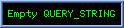1-potentials
A 1-potential (aka. a four-potential) for Â3,1 flow mp is sometimes presented as a 1-vector Â3,1 field satisfying both
Ñp¿ ap = 0     and     Ñp2 ap = - mp .

More generally we postulate a 1-vector ap = Ap + c-1Vpe4 satisfying ÑpÙap = -fp for irrotational pure bivector 2-field fp. so that
mp = m-1 Ñpfp = -m-1 Ñp(ÑpÙap) = -m-1 Ñp¿(ÑpÙap) = -m-1 (Ñp2ap - Ñp(Ñp¿ap) ) .
The tighter Lorentz condition Ñp¿ap = 0 provides Ñp2ap = -mmp .

A 1-potential is said to be e4-static or static if Ðe4ap » 0 " p so that Ðe4 derivatives vanish. Physicists also sometimes refer to potentials of the form ap = a4pe4 as static. Such an ap is seen as entirely nonmagnetic (ie. electrostatic) by e4-observers.
Physicists refer to 1-potentials of the form ap = a(|^e4(p-c)|) as central so that a static central potential has the form ap = a4(|^e4(p-c)|)e4 , a 4D 1-potential formed by using a 3D 0-potential as the e4 coordinate of an otherwise zero 1-vector. A notable example is the Lienard-Wiechart 1-potential ap = |e4Ùp|-1 e4 = |P|-1 e4     (where p=P + p4e4) which has Ñpap = -|P|-3 Pe4 = -|P|-4 (PÙe4)~     and     Ñp2ap = 0 (since N=4).

If the e4-magnetic (within e123) component bp of a Faraday 2-field fp = ep - bp is non-solenoidal ( Ñp¿bp ¹ 0) at p we say that p is a magnetic source. Other than at such a source, Ñp¿bp = 0 and we can construct a 3D 1-potential 1-vector Ap with Ñp[e123]Ap = Ñp[e123]ÙAp = bp .
We then have ep = fp + bp     =   -Ñpap + Ñp[e123]Ap   =   -(Ñp[e123]a4)e4 - Ñp[e4]Ap   =   -(Ñp[e123]a4)e4 - e4(Ðe4Ap)
[  Rindler[7.49] has Ñp2ap=4pc-1 jp in Â1,3, Lounesto[13.1] has Ñpap = -fp in Â3,1 ]
A flow satisfying Ñp2mp = -lmp "meets" its own 1-potential.

If fp is a scalar field satisfying Ñp2fp = lfp then 1-vector fpup satisfies Ñp2fpup = lfpup for any monogenic 1-vector up satisfying Ñpup=0 such as up = u or up = p|p|-N .
If fp satisfies Ñp2 fp = lfp (such as f(x)=f(|x|) where f"(r) +(N-1)r-1f'(r) = ±lf(r) according as x2 is ±) then fpd solves the Laplace Equation for any p-independant d.
If f(r) satisfies f"(r) + (N-1)r-1f'(r) - (N-1)r-2f(r) = lf(r) then f(|x|)x~ satisfies Ñx2 f(|x|)x~ = l f(|x|)x~ .
[ Proof : Ñ(f(|x|)x~) = (Ñf(|x)|)x~ + f(|x))Ñ(x~) = ±f'(r)x~2 + f(r)(N-1)r-1 = f'(r) + f(r)(N-1)r-1
Ñx2f(r)x~ = ±f"(r)x~ ±f'(r)(N-1)r-1x~ -/+ f(r)(N-1)r-2x~ = ±(f"(r) + (N-1)r-1f'(r)-(N-1)r-2f(r))x~  .]

Thus we seek f(r) solving f"(r) + (N-1)r-1f'(r) + (l - (N-1)r-2)f(r) = 0 .

Bivector Force Fields
If p'(t)2 is constant then Wp = -p"(t)p'(t) = -p"(t)Ùp'(t) is a pure plussquare bivector . In electrodynamics physicists infer from a trajectory curve solving p" = Wp.p' the existance of a pervading Faraday electromagnetic  bivector force field quantifiable (along p(t)) as
fp(t) = (m0/q0)W(t) + b2(p(t)) where arbitary spacelike 2-blade b2 Î p'* cannot be quantified (deduced) from the trajectory ; and proper mass m0 and proper charge q0 are two scalars "associated" with the particle.
The minus sign in Wp = -p"(t)p'(t) is necessary to accomodate the negative signature of p'(t) . It does not arise in Â1,3 timespace.

If we instead use p" = (Wpp')<1> we can set Wp = Wp plus an arvbitary <0;4>-vector.

In the case of the helical trajectory p(t) = (½w(1-R2w2)t e21)§(e3) we have p"(t)p'(t)   =   -(1-R2w2)-3/2w2R Rt§(Rwe21 + e41)     so (p"(t)p'(t))2   =   (1-R2w2)-2w4R2 .

Bivector field fp decomposeses into electrostatic and magnetic components = c-1ep + bp where bp = Be123-1 lies in Îe123 and e=Ee4 has e4Ùep=0

We "incorporate the local medium" by defining electric displacement Dp = epEp and magnetic intensity spacial bivector hp = mp-1bp where linear dilectrics ep and mp-1 are unitless geometric parameters representing the medium. Scalars in the case of a isotropic medium ; p-independant in a (spaciotemporally) homogeneous medium in which case they are known as the permitivity and pemeability constants respectively.   Units can be chosen to make ep = mp = 1 in a "vacuum". In S.I. units we have
e0 = (2hca)-1Qe-2 = (hc)-1(2p) » 8.8541878176×10-12 F m-1 = (4p)-1 where one farad = 1 F=1 C V-1 = 1 m-2 kg-1 s4 A2 = 1 m-2 kg-1 s2 C2 = 10-7c2 m ; and m0 = 4p×10-7 H m-1 = 4p where one henri = 1 H = 1 m2 kg s-2 A-2 = 1 m2 kg C-2 = 107 m .

Bivector field gp = cDpe4 - Hpe123 = cepep - mp-1bp provides a geometric analog of the Minkowski tensor form of the Maxwell equations
Ñp¿gp = jp    ;     ÑpÙfp = 0
which, if gp = m-1fp for some p-independant geometric linear "dilectric" multiplier m-1,  are the 1 and 3 grade components of the single geometric Maxwell equation
Ñpfp   =   Ñp¿fp   =   m jp .

Splitting the "dilectric" into e4-relative scaling components feels nonrelativistic, and it is more natural to replace gp = cepep - mp-1bp with gp = mp(fp) where mp() is a 2-tensor transformation mapping bivectors to bivectors, point-dependant except in a homogenous medium. [ Maxwell's original equations were Ñ×H = J + D/t    ; Ñ×E = - B/t    ; Ñ.D = r    ; Ñ.B = 0     where H,E,D,and B are spacial 3D 1-vectors in e4*   ]
It is thus natural to regard bivector force field fp as more fundamental than the flow jp = mp-1 Ñp¿fp , which is fully defined by fp and    satifisfies "conservation law" Ñp¿jp=0 as an inevitable consequence of its construction.
If the 1-potential ap satisies Ñp¿ap=0 then we have Ñpap=-fp and hence
jp   =   mp-1 Ñp(fp)   =   -mp-1 Ñp2 ap   =   -mp-1 Ñp¿(ÑpÙap)     with mp-1=m-1 a point-independant scalar in an isotrophic linear medium. . [  In Â1,3 we have Ñp2ap = mjp ]

In a classical vacuum jp=0 and so Ñpfp = 0 which has pure Â3,1 bivector circularly polarised wave solution
fp = ak((k*p)e1234)     for any null 1-vector k and 1-vector a normal to k where a º ea denotes exponentiation. 4-blade e1234 is available as a commuting i here because fp is even.
Picking a frame with k=w(e3+e4) we observe that ke34 = k so that k((k*p)e1234) = k((k*p)e12) and we can express our solution as
fp = a(e3+e4)((w(e3+e4)*p)e12)   =   re1(e3+e4)((q+(w(e3+e4)*p))e12)   =   re1(e3+e4)((q+(w(e3+e4)*p))e1234)     where a = re1eqe12 is an arbitary 1-vector .
We say fp has scalar magnitude r>0, frequency w, and phase q. w>0 is known as right-circular polarisation whereas w<0 gives left-circular polarisation.

More generally fp = ((k*p)ab) kA has Ñpfp=0 for any null 1-vector k and constant A, b if a¹0 and k commutes with b.
Thus if Fp is any solution to ÑpFp = lFp that commutes with null 1-vector k then Fpk((p¿k)-2j) for integer j³1 is also solves ÑpFp = lFp and by taking j sufficiently large we can ensure Fp is normalisable over nullcnes. by right multiplication by k((k*p)-2jb) for integer j³1, null 1-vector k, and any b commuting with k, eg. b=1 to obtain another solution .

Such a bivector field fp is perceived by a (4-frame) observer E as
f(t) = ^e4(t)*(f(p(t))) + ¯e4(t)*(f(p(t))) = ^e4(t)*(f(p(t))) - (¯e4(t)*(f(p(t)))*)* = EE(p(t))e4 - BE(p(t))e123
where e123 1-vectors EE(p(t)) º ^e4(t)*(f(p(t)))e4-1 and BE(p(t)) º¯e4(t)*(f(p(t))) e123-1 are known as the relative electric field and the relative magnetic field respectively.
Relative magnetic fields are more naturally represented by e4* bivector bp º bE(p(t)) = Bpe123 giving fp = c-1ep - bp but such decompositions are frequently more confusing than useful and it is better to consider the unified field fp.

In particular, ¯e4*(p") = (q0/m0) ¯(f,e4*) = (q0/m0)eA so a charge at rest is accelerated only by its relative electric field .

Path tsphere Intersections
Suppose we have a particle on a timelike worldline Q={q(t) : tÎ[t0,t1]} . For a given point p, where does the path Q intercept geometrically noninvertible L-p or invertible O-p,h0. It can cross L-p or O-p,h0 at most once if q'(t) is always strictly timelike , and if we assume the particle to have existed for long enough (t0 suffiently "early") it must cross somewhere so O-p,h0 Ç Q is a 1-horoblade correseponding to a   single embedded event q(tp).
Let t be the natural parameterisation so that v(t) = vp º q'(t) is unit timelike " tÎ[t0,t1]. We thus have a scalar field tp=t(p) returning the particle-clock time of the unique event of particle traversal of the rear nullcone L-p.
Define null or backward timelike 1-field  s(p) º sp º  q(t(p))-p with sp2=-h02 and unit timelike 1-field vp º v(tp) .

Euclidean preconceptions might lead us to expect Ñp t(p) to be parallel to vp=v(t) but instead we have
Ñp tp = sp (sp¿vp)-1     giving sp¿(Ñptp) = 0    ;     and vp¿(Ñptp) = 1 .
[ Proof : Ñp(spÑ¿sp)   =   Ñp((q(t(p))-p)Ñ¿sp)     =   Ñp(q(t(p)Ñ¿sp) - Ñp(pÑ¿sp)   =   (Ñpt(p))vp¿sp - sp
But Ñp(sp2)=Ñp(h02)=0 so (Ñpt(p))vp¿sp = sp. [ GAfp 7.84 ]  .]

The undirected scalar-funneled chain rule gives
Ñp sp = Ñp(q(tp)-p) = (Ñptp)vp - N = (sp¿vp)-1 spvp - N (sp¿vp)-1 spvp - N .
Ñp vp = (Ñptp)vp' = (sp¿vp)-1 spvp' .

Ñp (sp¿vp)   =   -(sp¿vp)-1 ( sp(spÙvp') + vp(spÙvp)) = -½(sp¿vp)-1 ( vpspvp + sp2vp' - vp2sp - spvp'sp)
[ Proof : From a¿(bÙc)=(a¿b)c-(a¿c)b we have
Ñp(sp¿vp)    = (sp¿Ñ)vpÑ + (vp¿Ñ)spÑ - sp¿(ÑpÙvp) - vp¿(ÑpÙsp) = Ðspvp + Ðvpsp - sp¿(ÑpÙvp) - vp¿(ÑpÙsp) .
The directed scalar-funnelled rule   ÐAF(g(X)) = F'(g(X))gÑ(A)=(A*(Ñxg(X))) F'(g(x)) enables verification that Ðvpsp = Ðspvp = 0   so we have
Ñp(sp¿vp)    = - sp¿(ÑpÙvp) - vp¿(ÑpÙsp) = - sp¿(Ñpvp)<2> - vp¿(Ñpsp)<2>
= -(sp¿vp)-1 ( sp¿(spÙvp') + vp¿(spÙvp)) = -(sp¿vp)-1 ( sp(spÙvp') + vp(spÙvp))
= -(sp¿vp)-1 ½( sp(spvp'-vp'sp) + vp(spvp-vpsp))  .]

Nonnegative (since vp is forward and sp backward) scalar sp¿vp is physically important as the spacial distance (as percieved by the particle at qp) from qp to p. It vanishes for null sp only when vp is also null and parallel to sp, , whereupon sp¿(vpÙvp') vanishes. If sp=-gU(e4+Up) is backward timelike,  sp¿vp is minimised by timelike vp =gV(e4+Vp) as gUgV(1-Vp2Up2) where Up is opposite parallel to Vp.

Propagated Lienard-Wiechart Potential
Suppose a timelike (or null) trajectory particle at qp with unit (or null) four-velocity vp and acceleration vp' instantaneously generates a timelike (or null) 1-potential ap = f(sp¿vp) vp where f: Â®Â. We have
fp   =   -Ñp ap   =   -(sp¿vp)-1 ( f'(sp¿vp)( -sp(spÙvp')vp + vp2(spÙvp)) + f(sp¿vp)spvp' )
[ Proof : Ñp(f(sp¿vp)vp)   =   (Ñpf(sp¿vp))vp +  f(sp¿vp) (Ñpvp)   =   f'(sp¿vp)(Ñp(sp¿vp))vp + f(sp¿vp)(sp¿vp)-1 spvp'
= -f'(sp¿vp)(sp¿vp)-1 (sp(spÙvp')+vp(spÙvp))vp + f(sp¿vp)(sp¿vp)-1 spvp' =   (sp¿vp)-1( f'(sp¿vp)( -sp(spÙvp')vp+vp2(spÙvp)) + f(sp¿vp)spvp') .]

Setting f(sp¿vp) = a(h0-(sp¿vp)2) for some p-independant fixed scalar "mass" or "charge" or "ammount" a we have
fp = -Ñpap =   -(sp¿vp)-1 ( -½((h0-(sp¿vp)2)-3/2  (sp¿vp)( -sp(spÙvp')vp + vp2(spÙvp)) + (h0-(sp¿vp)2) spvp' )
=   -(sp¿vp)-1 (h0-(sp¿vp)2)-3/2( -½(  (sp¿vp)( -sp(spÙvp')vp + vp2(spÙvp)) + (h0-(sp¿vp)2)spvp' )

For f(sp¿vp) = a(sp¿vp)-1 corresonding to a Lienard-Wiechart potential a |^vp(sp)|-1 vp for null sp we have
ap = a (sp¿vp)-1 vp = -a (¯vp(sp))-1 and
fp   =   -Ñp ap   =   a (sp¿vp)-3( vp2(spÙvp) + (spvp')×(spvp) )
[ Proof : Ñp((sp¿vp)-1)vp)   =   (Ñp((sp¿vp)-1))vp +  (sp¿vp)-1 (Ñpvp)   =   -(sp¿vp)-2(Ñp(sp¿vp))vp +  (sp¿vp)-2 spvp'
= (sp¿vp)-3(½( vpspvp + sp2vp' - vp2sp -spvp'sp)vp + (sp¿vp) spvp')
= ½(sp¿vp)-3( vp2vpsp + sp2vp'vp - vp2spvp - spvp'spvp + (spvp+vpsp)spvp')
= (sp¿vp)-3( sp2(vp¿vp') + vp2(vpÙsp) + (spvp)×(spvp') )   =   (sp¿vp)-3( vp2(vpÙsp) + (spvp)×(spvp') )  .]

Both terms of Ñpap are pure bivectors so Ñp¿ap = 0, as required of a 1-potential, for any trajectory q(t). Note that
(spvp')×(spvp)   =   (spvp')(spvp) - (spvp')¿(spvp)     =   (spvp'sp)vp - (spvp'sp)¿vp     =   (spvp'sp)Ùvp   =   -(spvpsp)Ùvp'

When n2=-1 we have -nxn = ¯n(x) - ^n(x) so (spvp')×(spvp) =   -((¯sp(vp')-^sp(vp'))Ùvp =   ((¯sp(vp)-^sp(vp))Ùvp' .   =   (spvp')(spvp) - (spvp'spvp)<0>     =   (spvp')(spvp) + ((¯(vp',sp)-^(vp',sp))vp)<0>     =   (spvp')(spvp) + (¯(vp',sp)-^(vp',sp))¿vp   =   (spvp')(spvp) + (¯(vp,sp)-^(vp,sp))¿vp' .
Since fp acts via up' = (qm-1) fp¿up we can add a scalar part to fp provided we make the ¿ in Maxwells equation  Ñpfp   =   Ñp¿fp   =   mp jp explicit. It is thus natural to set <0;2>-field
¦p   =   a (sp¿vp)-3( vp2spvp) + (spvp')(spvp) )   =   a (sp¿vp)-3spvp'spvp for null vp.

For null sp, the  (spvp')×(spvp) = (spÙvp')×(spÙvp) term is equal to -2sp(vp'Ùvp)sp = -2spWpsp = -4(sp¿Wp)sp = -4(sp¿Wp)Ùsp     where Wp=-vp'Ùvp. [  by the null reflected 2-blade rule sabs = s(aÙb)s = sbsa - sasb ] and we obtain
fp   =    avp2(sp¿vp)-2 (spÙvp)~   + ½a(sp¿vp)-3 spWpsp for the null-propagated Lienard-Wiechart field.

If vp is also null we have the purely radiative fp   =     ½a(sp¿vp)-3 spWpsp .
Thus a charged nonaccelerating null-trajectory particle emmits no field. Acceleration (vp'¹0 generates a tightly pulsed r-1 field that tends to alter orbital momentum about the wordline (Biot-Savart law) . Timelike traversal incurrs an r-2 spÙvp field that never vanishes but approches 0 as (sp¿vp) ® ¥ .
In the electromagnetic ("current") case, we have a = (4pe0)-1q where q is the "fixed charge" of the particle. The Faraday bivector at p is given by -ÑpÙap = -Ñpap and so contains an "invere square" Coloumb boost , central for a static (vp=e4) wordline ; and a mostly tangential "reciprocal law" radiation ( Bremstrahlung, synchrotronic) null boost due to the particle acceleration vp'. In the gravitic (momentum) case these are regarded as an inverse square "Newton" boost and a reciprocal "gravitomagnetic" boost.
For a null worldline with vp2=0 generating a null Lienard-Wiechart 1-potential, the inverse square term vanishes and we are left only with the radiation term. The unbounded |vp¿sp|-3 factor is infinite only when (vp'Ùvp)sp=0 so such a null 4-potential is physically plausable.

If we allow a=ap=a(t(p)) to vary with t we aquire an additional (Ñap) (sp¿vp)-1 vp term in Ñpap which is expressable as (a'p) (sp¿vp)-2 spvp and is not a pure bivector since spvp has a scalar part. Thus if a partcle varies its charge or mass, the resultant 1-potential has nonzero point divergence.

When sp2=h02 ; vp2=0 we have fp   =   -Ñp ap   =   a (sp¿vp)-3 (spvp')×(spvp)   =   a (sp¿vp)-3 ((spÙvp')(spÙvp))<2> and can set
up'   =   (sp¿vp)-3 ((spÙvp')(spÙvp)up)<1> .

Field due to straight worldine
When vp'=0 we have fp = a (sp¿vp)-3 vp2(spÙvp) which is 0 for a vp2=0 null worldine and -a (sp¿e4)-3(spÙe4) for the vp2=-1 timelike worldine {te4 : t Î [-¥,¥] } .
For null propagation the field at p=X+te4 for XÎe123 is the the inverse square Coulomb field ((X-|X|e4)¿e4)-3(X-|X|e4)Ùe4  = |X|-2(Xe4)~ .
For sp2 = ±h02 and |X|2 > h02 we have sp=X-(X2 -/+ h02)½e4 and field -a(X2 -/+ h02)-3/2XÙe4 closely approximating the Coloumb field for |X| >> h0.

Instananeous Field due to helical worldine
Consider a particle orbitting 0 at distance R within the e12 plane having proper time formulation
q(t) = (½wgt e21)§ ( Re1 + tge4) where g = |1-R2w2| and |Rw| < 1.
We may be particularly interested in R = ¼hp-1 m-1c-1 (ie. ½m-1 in natural units).

At t=0 we have q'(0)= g(Rwe2 + e4) ; q"(0)   =   -(1-R2w2)-1w2Re1 ; and q'(0)q"(0)   =   -g3w2R(Rwe21 + e41) .

The interesction of a helix and  a null cone is algebraically complex but can be numerically established, eg. via a binary search on t, relatively straightforwardly. The e4-instantaneous field takes the form of a fixed width and span spiral containing a pulse of strength in proportion to r-1 . If we integrate the field over one period at a fixed spacial location P (from P to P + 2pw-1e4 say) then, apart from in a localised "ring" region with r»1 , the e12 and eqe4 componensts average to zero while the radial ere4 components averages to uniform radial field proportionate to r-2.
Such integrations are both computationally problematic (the spiral wavefront is travelling at at supralight speeds for r>1 and "sweeps over" a test point very rapidly, making the integration one of a quasi-discontinuous Dirac-delta like spike) but also physically suspicous since a test partcle will actually move with the field to some degree, The radial erÙeq pulse,  for example, will ted to slightly increase r via a tangental kick, and so the "restoring" effect will be reduced due to the r-1 factor.

 Retarded Lienard-Wiechart Field due to unit radius helix at e4-time t=0 when particle is at e1. 10 × 10 square area radially scaled by r over r>1 ; logged and clipped at bright red (negative) and bright green (positive). Bottom left quadrant shows erÙe4 "Coulomb" component , bottom right shows eqÙe4 "Biot-Savart" compoent , top left shows erÙeq=e12 "magnetric moment" component. Top right shows (nonphysical?) scalar part.     Note how field apparently changes sign due to the narrowing of the central peak, and contracts slightly as g increases from 1¼ (slow) to ¥ (lightspeed).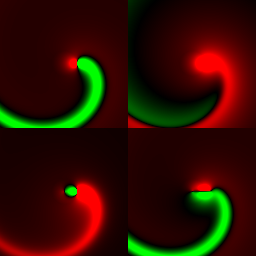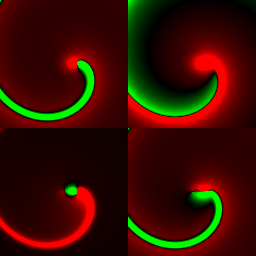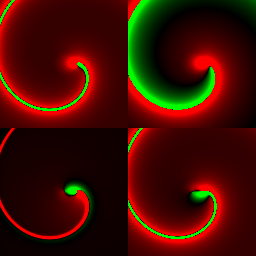g=5/4 10×10 region g=3/2 10×10 region g=2 10×10 region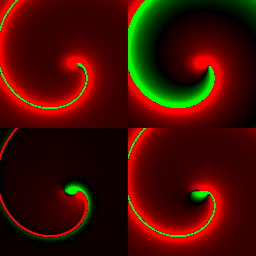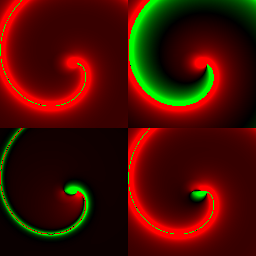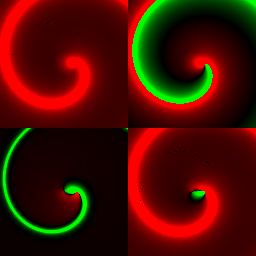g=2 1/4 10×10 region g=3 10×10 region g=¥ 10×10 region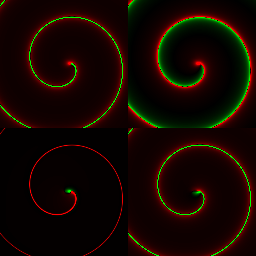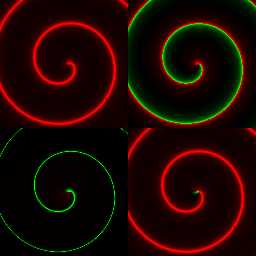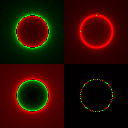g=2 27 sample averageg=4 27 sample average g=2 20×20 region g=¥ 20×20 region T-averaged g=2 field. Fine structure is computional artifact from sampling timestep 2-7T ie. at roughly the Treiman timestep.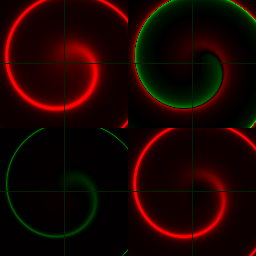g=2 20×20 region g=¥ 20×20 region 20×20 g=¥ timelike seperation We now plot spacelike unit helices (R=1, w > 1), Retaining 10×10 area we scale all but the er4 field by w-1 .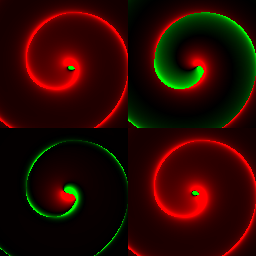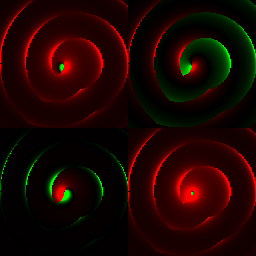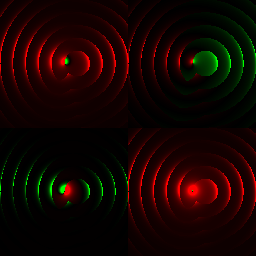w=2-1p w=p w=2p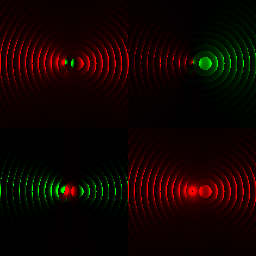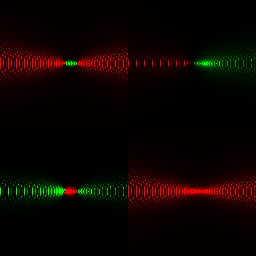w=22p w=24pClick to animate.Click to animate.Click to animate.Click to animate. Orbitting common centre   phase p 20×20 unit seperated centres same spin phase p 20×20 unit seperated centres opposite spin phase p 40×40 unit seperated centres opposite spin phase p

The spin in the trajectory thus introduces an oscillation in the field. Rather than being smmothly varying over time and space, the field is "bunched" into a pulse with a profile that changes sign. The pulse is preceeded by an opposite torque that serves to draw a test charge into a repulsive wave and away from an attractive one. This will tend to amplify repulsive effects compared to attractive ones.

Averaged Field due to helical worldine

Integrating the field at spaical point P over the helical period (2p)-1w gives the net imupulse applied by a single field pulse to a test charge fixed at P. This is somewhat unphysical since a free test charge that "rides" the field and moves slightly during the pulsetime may experience a significantly different impulse due to being "brushed aside from" or "drawn in to" the main pulse. Nonetheless, if we think of a compartively heavy test charge slow to respond to the field, summing the field at P over e4¿p Î[t,t+(2p)-1w] provides a reasonable indicator of the effective field at p.
Analytically, such integerations are nontrivial. Computationally, they are problematic in that the integrated field is exceptionally bunched making naive applications of Simpson's Rule likely to "miss" the pulse and give high errors. Adpative refinement methods are required and, since each field evaluation involves a worldline intersection, the calculation load is significant.

Self Interaction

Timelike self interaction of null helix

For timelike sp2=-h02 we have the possibility of self-interaction. Recalling that vp and Wp describe the matter at p+sp, and letting up and Kp denote the matter at p we have up'=up¿Kp = up¿((qm-1)fp)   =   (qm-1) up¿fp where
Kp =-up'up   =   g3w2R Rt§(Rwe12 + e14)   =   -g3w3R2e12 - g3w2R Rt§(e14) .

Suppose p=Re1 and sp = -2kpw-1e4 with sp2 = -4(kp)2w-2 so that vp = up = gRwe2 + ge4     ;     vp' = up' = -g2w2Re1 .
It follows that, at p=Re1, fp   =   a (sp¿vp)-3( vp2(spÙvp) + (spÙvp')×(spÙvp) )   =   a (2kpw-1g)-3 ( -2kpgRe24 -4(kpg)2wR2ge12 )
[ Proof : sp¿vp =   (-2kpw-1e4)¿(gRwe2 + ge4)   =   2kpw-1g
spÙvp     =    2kpgRe24        ;     spÙvp' =  (-2kpw-1e4)Ù(-g2w2Re1)   =    -2kpwRg2e14 .
(spÙvp')×(spÙvp)   =   -2kpwRg2e14 × 2kpgRe24   =   -4(kpg)2wR2ge12 and result follows.  .]

When vp2=0 (so Rw=g=1) we have fp   =   - a(2kpw-1)-3 4(kp)2R e12   =   -a(2kp)-1w2 e12 and so (qm-1)fp¿up   =   -(qm-1)a(2kp)-1w2 e1 where m is the "inertial mass" of the worldline.
This is equal to the desired up' = -we1 if (qm-1)a(2kp)-1w = 1   , ie. if m = (2kp)-1aq w   =     (2kp)-1aq (2ph0-1)   =     k-1aq h0-1 .
It is conventional to set a = q(4pe0)-1 giving m   =     k-1q2 (4pe0)-1 h0-1   =   a k-1 h0-1  where a = q2 (4pe0)-1 [   q2 (4pe0 (2p)-1hc)-1 in unnatural units ] is the fine structure constant.

Hence choosing a particluar effectation seperation h0 we have a selfinteracting null helical equilibrium with R=(2p)-1h0 , w = ± 2ph0-1 , and period h0 provided the inertial mass attributed to the particle is m   =     k-1a h0-1 for some integer windage number k , the worldine being influenced by itself k periods back  .

Setting q=1 , our model is thus a null worldine radiating potential ap = a (sp¿vp)-1 vp = (4pe0)-1 (sp¿vp)-1 vp and responding to the induced field fp as up' = (qm-1) fp ¿ up   =   k(4pe0)h0 fp ¿ up.   =   -kh0 (Ñp((sp¿vp)-1vp)) ¿ up.

If R >> 2ph0 there will be some time t such that sp = (1-R cos(wt))e1 - R sin(wt)e2 - te4 has
sp2 =((½wt)-1)2 - t2 = R22(1- cos(wt))   - t2 = -h02 and |spvp| will tend to be small so fp will be large and tend to increase the acceleration, decreasing the radius of the helix. Once a null streamline deviates from inertial, perhaps by the influence of a seperate worldine, then it will "capture its own past" and coil into a tight self-maintaining helix. .
We then have sp¿vp = ( (1-R cos(wt))e1 - R sin(wt)e2 - te4)¿(e2+e4) = - R sin(wt) + t ;
spÙvp = ( (1-R cos(wt))e1 - R sin(wt)e2 - te4)Ù(e2+e4) = (1-R cos(wt))e1Ù(e2+e4) + (t-R sin(wt))e24   ;
and spÙvp' = -( (1-R cos(wt))e1 - R sin(wt)e2 - te4) Ù _ome1 = - sin(wt))e12 - te14 .

(spÙvp')×(spÙvp) = - ( sin(wt))e12 + te14) × ((1-R cos(wt))e1Ù(e2+e4) + (t-R sin(wt))e24)   ; -
 Field due to unit radius null and timelike propagated null helix at e4-time t=0 when particile is at e1.   10×10 region.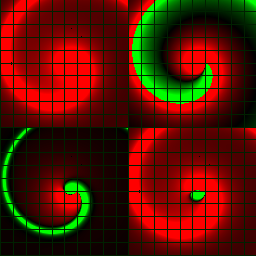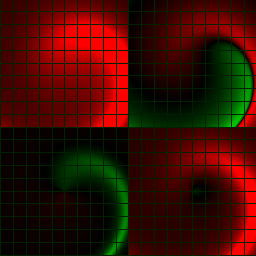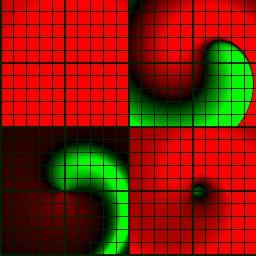g=¥ null seperation as above but brighter colouring g=¥ timelike seperation Weaker and wider field g=¥ timelike seperation with increased brightness.

More generally a helix will "experience itself" at p(0)=Re1 as the field it radiated at p(-t) where t solves (p(t)-Re1)2=h02 Û R2((1- coswgt))2 + sinwgt)2) - g2t2 = h02 Û 2R(1- cos(wgt)) - g2t2 = h02 Û 2R(1- cos(wgt)) - g2t2 = h02 Û 2R - (¼w2R + 1)g2t2 - 2Råj=2¥ (wgt)2j(2j)!-1   =   h02 .

More generally still, we have the field at Re1 dues to a helix p(t)= R((wgt+f)e12)e1 + gte4 where f is a phase angle, providing self interaction when f=0.

Null self interaction of spacelike helix

For a null-seperated self-interaction with sp2=0 we require a spacelike helix with vp2=1. Suppose p=Re1 and sp = -2Re1 - kpw-1e4 with sp2 = 4R2-k2p2w-2 . For sp2=0 we require Rw = ± ½kp .

Set g = gk º ((½kp)2   - 1) and hk º wRgk = ±kp)((½kp)2 - 1) with hk2-gk2 = 1.
vp   =   -gRwe2 + ge4   =   -hke2 + gke4     ;     vp' = g2w2Re1 = R-1hk2e1
up = gRwe2 + ge4   = hke2 + gke4     ;     up' = -g2w2Re1 = -R-1hk2e1 .

sp¿vp =   (-2Re1 - kpw-1e4)¿(-gRwe2 + ge4)   =     kpw-1g
spÙvp =   (-2Re1 - kpw-1e4)Ù(-gRwe2 + ge4)   =   2R2gwe12 - kpgRe24 - 2Rge14   =   Rg(2Rwe12 - kpe24 - 2e14)
spÙvp' =  (-2Re1 - kpw-1e4)Ù g2w2Re1   =    kpwRg2e14 .
(spÙvp')×(spÙvp)   =   kpwRg2e14× Rg(2Rwe12 - kpe24)   =   kpwR2g3(2Rwe24 - kpe12)

These yield fp   =   a (sp¿vp)-3( vp2(spÙvp) + (spÙvp')×(spÙvp) )   =   -/+ a ½(kp)-2w2gk (   2e14 + (kp)(1-2hk2)(-e12±e24) )
[ Proof : a (kpw-1g)-3 ( Rg(2Rwe12 - kpe24 - 2e14) + kpwR2g3(2Rwe24 - kpe12))
=   a (kp)-3g-2w3 R (2Rwe12 - kpe24 - 2e14 + kpwRg2(2Rwe24 - kpe12))
=   ± a ½(kp)-2w2g-2 (±kpe12 - kpe24 - 2e14 ± ½(kp)2g2(±kpe24 - kpe12))
=   a ½(kp)-2w2g-2 (kpe12 -/+ (kpe24 + 2e14) + ½(kp)2g2(±kpe24 - kpe12))
=   a ½(kp)-2w2g-2 (   -/+ 2e14 + (kp) (   (1-½(kp)2g2)e12 + (±½(kp)2g2 -/+ 1) e24 )
=   a ½(kp)-2w2g-2 (   -/+ 2e14 + (kp)(1-½(kp)2g2)(e12   -/+ e24) )
=   a ½(kp)-2w2g-2 (   -/+ 2e14 - (kp)(1-2hk2)(-e12   ± e24) )
=   ± a ½(kp)-2w2g-2 (   -2e14 - (kp)(1-2hk2)(-e12   ± e24) )
since (1-½(kp)2g2) = (1-½(kp)2((½kp)2-1) .  .]

Hence fp¿up   =   -/+ a ½(kp)-2w2gk (   2e14 + (kp)(1-2hk2)(-e12±e24) ) ¿ (hke2 + gke4)
=   -/+ a ½(kp)-2w2gk (   -2gke1 + (kp)(1-2hk2)(-hke1-/+hke4 -/+ge2) )
=   ± a ½(kp)-2w2gk (   2gke1 + (kp)(1-2hk2)(hke1±hke4 ±ge2) )

The e1 coordinate of up' = (qm-1)fp¿up is thus ± (qm-1)a ½(kp)-2w2gk (   2gk + (kp)(1-2hk2)hk) which equals the desired -g2w2R if

=   a ½(kp)-2w2g (   -/+ 2e14 - (kp)(1-2hk2)(e12 ± e24)) ¿ (±hke2 + gke4)
=   a ½(kp)-2w2g ( (±2 -/+ (kp)(1-2hk2)hk)e1 ± (kp)(1-2hk2)(gke2+hke4) )

Hence fp¿up   =    a (kpw-1g)-3 Rg ( Rw(2-(kpg)2)e12 + kp(2w2R2g2-1)e24 - 2e14) ¿ g(Rwe2 + e4 )
=    a (kp)-3w3Rg-1 ( (2+(Rw)2(2-(kpg)2)e1 + kpRw(1-2w2R2g2)e2 + kpRw(1-2w2R2g2)e4 )
=    a (kp)-3w3Rg-1 ( (2+(Rw)2(2-(kpg)2)e1 - kpRw(e2+e4) ) [  since (1-2w2R2g2) = g2(g-2-2w2R2) = -g2(w2R2-1) = -1 when |Rw| > 1 ]

Setting Rw = ± ½kp , g = gk = (2-2k2p2-1) so that   (2-(kpg)2) = ((½kp)2-1)-1(2(½kp)2-3) gives
fp¿up   =   a (kp)-3w2 (±½kp)gk-1 ( (2+¼(kp)2(2-(kpg)2)e1 -/+ ½(kp)2(e2+e4) ) a w2 (±½)gk-1 ( (2(kp)-2+¼(2-(kpg)2)e1 -/+ ½(e2+e4) ) .

The e1 coordinate of up' = (qm-1)fp¿up is thus (qm-1) a w2 (±½)gk-1 ( 2(kp)-2+¼(2-(kpg)2)) which equals the desired -g2w2R if
g2R   =   (qm-1) a ½gk-1 ( 2(kp)-2+¼(2-(kpg)2)) Û R = (qm-1) a gk3( ¼ + (kp)-2 - 1/8(kpg)2) .

Constructing the potential
The previous discussion constructed the potential at a given event p due to a worldline q(t) in accordance with null-seperated interactions. We considered only the "particle-instant" p+sp in the rear null cone L-p to contribute, perhaps by means of a virtual particle traversing a straight null path. Becuase we considered the matter at p+sp to be represented by the timelike v(t(p))=vp  it was natural to allow it to contribute a vp-static potential of zero-divergent magnitude (sp¿vp)-1 extended out from p+sp with vp considered constant. The higher derivatives like v'(t)=p"(t) did not directly contribute to the "instantaneous" potentiala at p, effecting it only indirectly by influencing sp

Given ap0   =   a (sp¿vp)-1 vp   =   a (sp¿jp)-1 jp   =   -a (¯vp(sp))-1     , it is natural to consider a small 4-simplex d4p at pÎ L-p0 as generating a potential a |d4pjp|((p-p0)¿vp)-1 vp = a |d4p|((p-p0)¿vp)-1 jp     at event p0 . If the simplex is positively orientated (|d4p| = d4pe1234-1) then we have a contribution a d4p jp((p-p0)¿jp)-1 i-1 .
Rather than integrate over noninvertable 3-surface L-p0 we might approximate with rear tsphere O--h0,p0 for small scalar eventgrain h0 >0 , centred at p0 and obtain
ap0   =   a ò Oh0,p0 |d3p| jp((p-p0)¿jp)-1     which is a sort of closeness-weighted average of jp.

This gives us a "growth" equation for jp
jp   =   -(4p)-1 Ñp2ap   =   -a(4p)-1 Ñp2 ò O-h0,p0 |d3p| jp((p-p0)¿jp)-1  º  Hp(jp)     where
Hp(y)  º  ò O-h0,p0 |d3p| yp((p-p0)*yp)-1 i-1 .
We can estimate jp for e4¿p=t0 by estimating Hp(y) from a reasonably dense sampling of jp for e4¿p<t0 , although we are forced to evaluate Ðe42 jp at e4¿p=t0 as a "approaching" rather than a "centred" gradient estimate.

Useful zero-divergent 1-fields

First we must consider 1-fields that are both nondivergent and solenoidal (Ñp¦(p)=0). These trivially satisfy Ñp2¦(p)=0 and are of little use for potentials since we typically require ÑpÙap nonzero.
¦(x) = Nax + (N-2)xa = 2(N(a¿x) - xa) has Ñx¦(x)=0 for any 1-vector a.

¦(x) = (x-x0)|x-x0|-N = (x-x0)~ |x-x0|1-N has Ñx¦(x)=0 since Ñp (x|x|-k) = (N-k)|x|-k .   However this undefined whenever (x-x0)2=0. Thus for N=2 ¦(x)= |x|-1 x~ is monogenic while for N=3 we need ¦(x)= |x|-2 x~ and in N=4 ¦(x)= |x|-3 x~ .

Define aa,b º aa,b(x)= aa,b(x2) x = x ea(x2)½ (x2)½b = ear rb x .
Then Ñx aa,b = Ñx( aa,b)x + N aa,b   =   (a aa,b-1+b aa,b-2) x2 + N aa,b   =   a aa,b+1+(b+N) aa,b     so that Ñx aa,-N = ar aa,-N

Consider ¦(x)=(-x3) = (-/+|x|3x~) according as x2 is ± . For x2>0, |¦(x)|®0 as x®¥ . For x2£0 ¦(x) = cos(|x|3) + sin(|x|3)x~ is unimodular with ¦(x)=1 over Lx.

Also available is (l(p-p0)*s)a for any point-independant null 1-vector s and poinmt-independant 1-vector a orthogonal to s since   Ñp(l(p-p0)*s)a = (l(p-p0)*s)(sÙa) and Ñp2(l(p-p0)*s)a = 0 .

For an e4-static 1-potential we have r-2 = r~ |r|-2 where r = ^e4(p-p0) well defined everywhere but along p0+te4.

Next : Gaussian Wave Packets

Glossary   Contents   Author
Copyright (c) Ian C G Bell 1999
Web Source: www.iancgbell.clara.net/maths or www.bigfoot.com/~iancgbell/maths
Latest Edit: 13 Jun 2014.